## RD Sharma Class 8 Solutions Chapter 6 Algebraic Expressions and Identities Ex 6.2

These Solutions are part of RD Sharma Class 8 Solutions. Here we have given RD Sharma Class 8 Solutions Chapter 6 Algebraic Expressions and Identities Ex 6.2

Other Exercises

Question 1.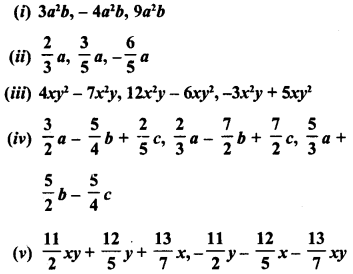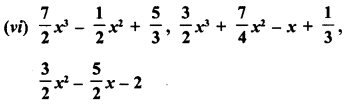Solution: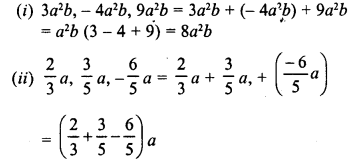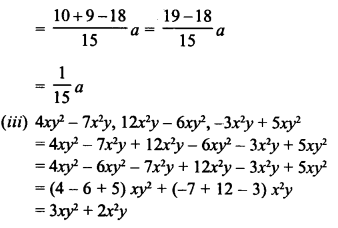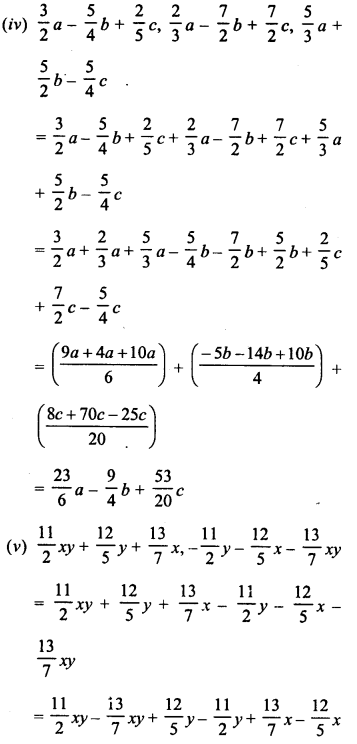Question 2.
Subtract:
(i) -5xy from 12xy
(ii) 2a2 from -7a2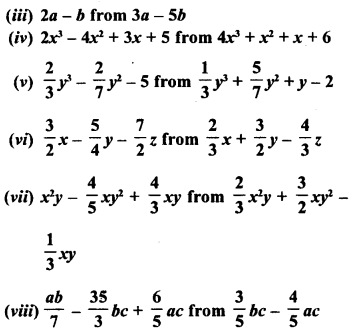Solution: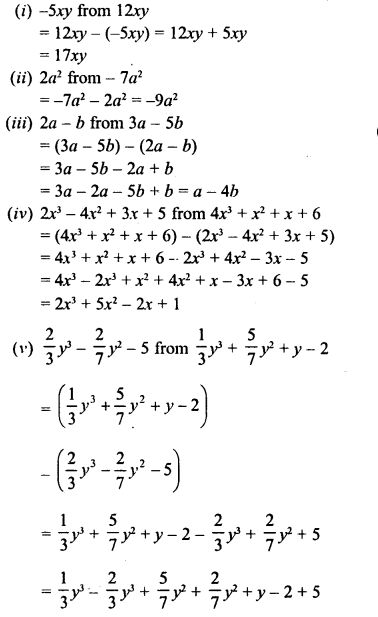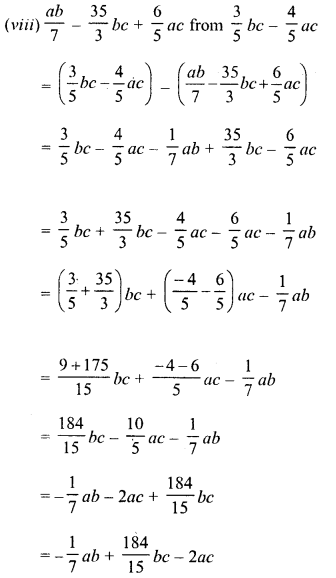Question 3.
Take away :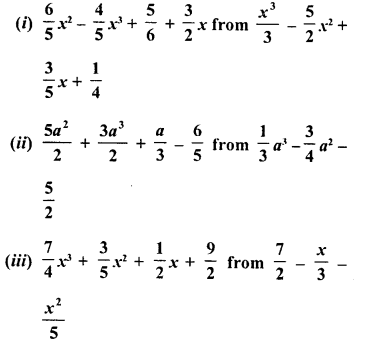Solution: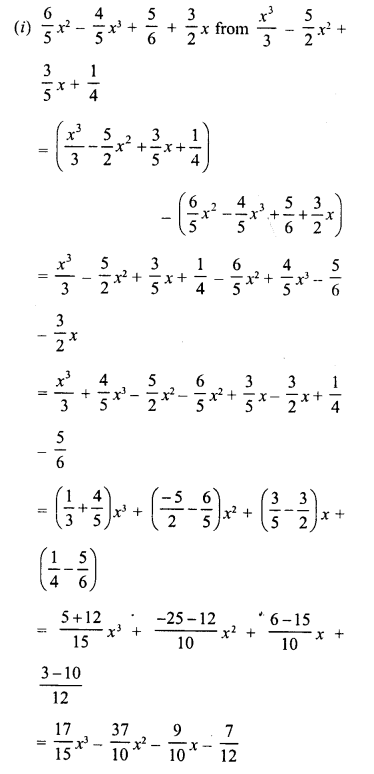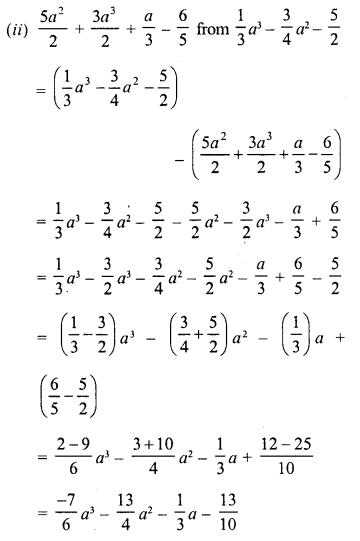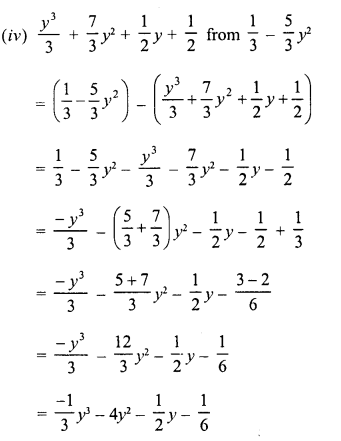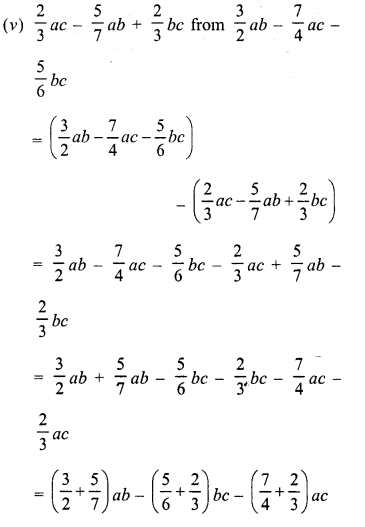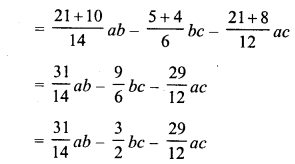Question 4.
Subtract 3x – 4y – 7z from the sum of x – 3y + 2z and – 4X + 9y- 11z.
Solution:
Sum of x – 3y + 2z and – 4x + 9y – 11z
= x – 3y + 2z + (- 4x + 9y – 11z)
= x – 3y + 2z – 4x + 9y – 11z
= x – 4x – 3y + 9y + 2z – 11z
= – 3x + 6y – 9z
Now (-3x + 6y – 9z) – (3x – 4y – 7z)
= -3x + 6y – 9z – 3x + 4y + 7z
= -3x – 3x + 6y + 4y -9z +7z
= -6x + 10y – 2z

Question 5.
Subtract the sum of 3l- 4m – 7n2 and 2l + 3m – 4n2 from the sum of 9l + 2m – 3nand -3l + m + 4n2.
Solution:
Sum of 9l + 2m – 3n2 and -3l + m + 4n2
= 9l + 2m – 3 n2 + (-3l) + m + 4n2
= 9l + 2m – 3n2 – 3l + m + 4n2
= 9l- 3l+ 2m + m – 3 n2 + 4n2
= 6l + 3m + n2
and sum of 3l – 4m – 7n2 and 2l +3m- 4n2
= 3l- 4m – 7n2 + 2l+ 3m- 4n2
= 3l + 2l – 4m + 3m- 7n2 – 4n2
= 5l -m- 11n2
Now (6l + 3m + n2) – (5l – m – 11n2)
= 6l + 3m + n2 – 5l + m + 11n2
= 6l – 5l + 3m + m + n2 + 11n2
= l + 4m+ 12n2

Question 6.
Subtract the sum of 2x – x2 + 5 and -4x – 3 + 7x2 from 5.
Solution:
5 – (2x-x2 + 5-4x-3 + 7x2)
= 5 – (2x – 4x- x2 + 7x2 + 5-3)
= 5 – (-2x + 6x2 + 2)
= 5 + 2x – 6x2 – 2
= – 6x2+2x+3
= 3 + 2x – 6x2

Question 7.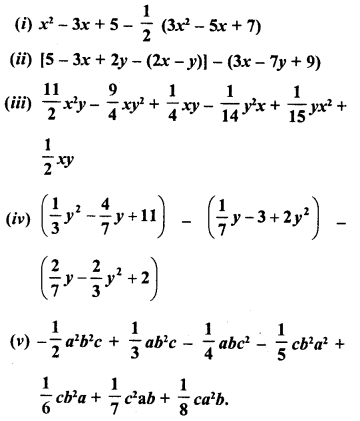Solution: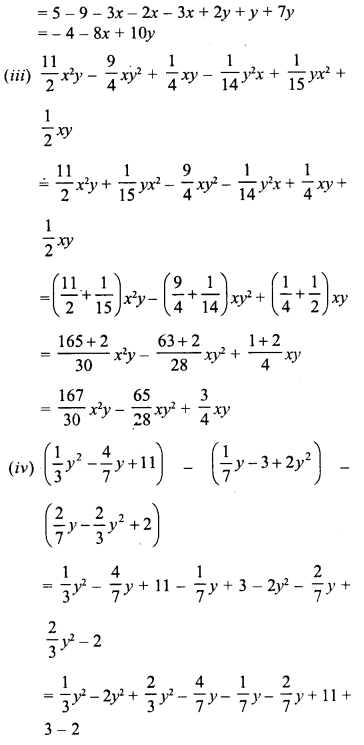Hope given RD Sharma Class 8 Solutions Chapter 6 Algebraic Expressions and Identities Ex 6.2 are helpful to complete your math homework.

If you have any doubts, please comment below. Learn Insta try to provide online math tutoring for you.